# 5.1 - 2 (2 points) Let f(x) Find the open intervals on which f is concave...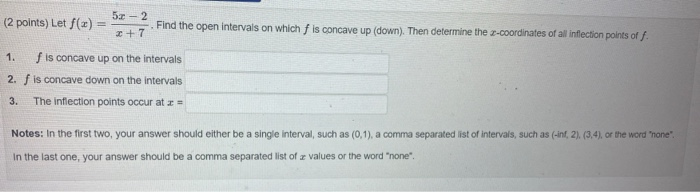5.1 - 2 (2 points) Let f(x) Find the open intervals on which f is concave up (down). Then determine the e-coordinates of all inflection points of 2 + 7 1. f is concave up on the intervals 2. f is concave down on the intervals 3. The inflection points occur at 2 - Notes: In the first two, your answer should either be a single interval, such as (0,1), a comma separated list of intervals, such as (-int, 2), (3,4), or the word "none". In the last one, your answer should be a comma separated list of values or the word "none".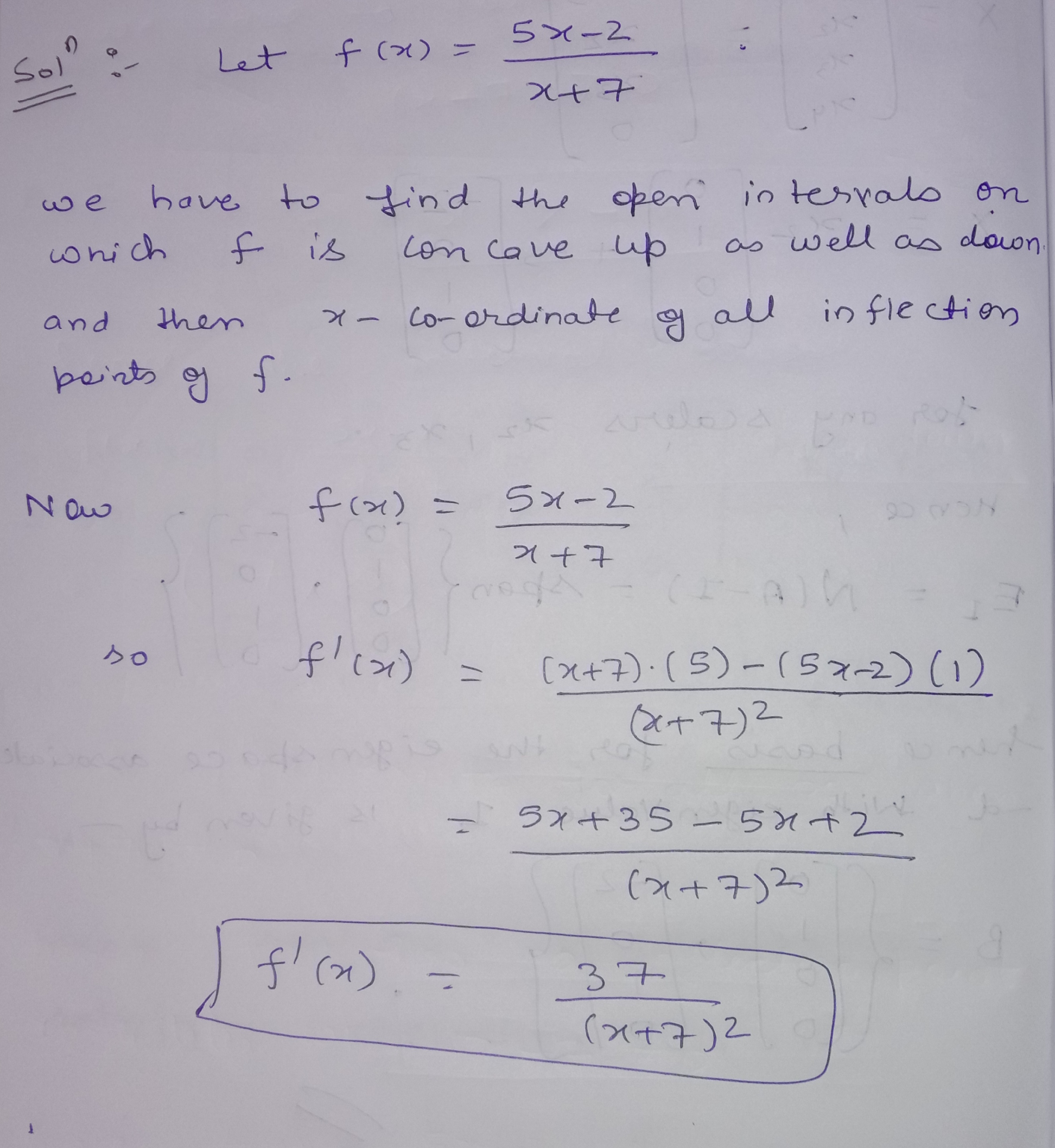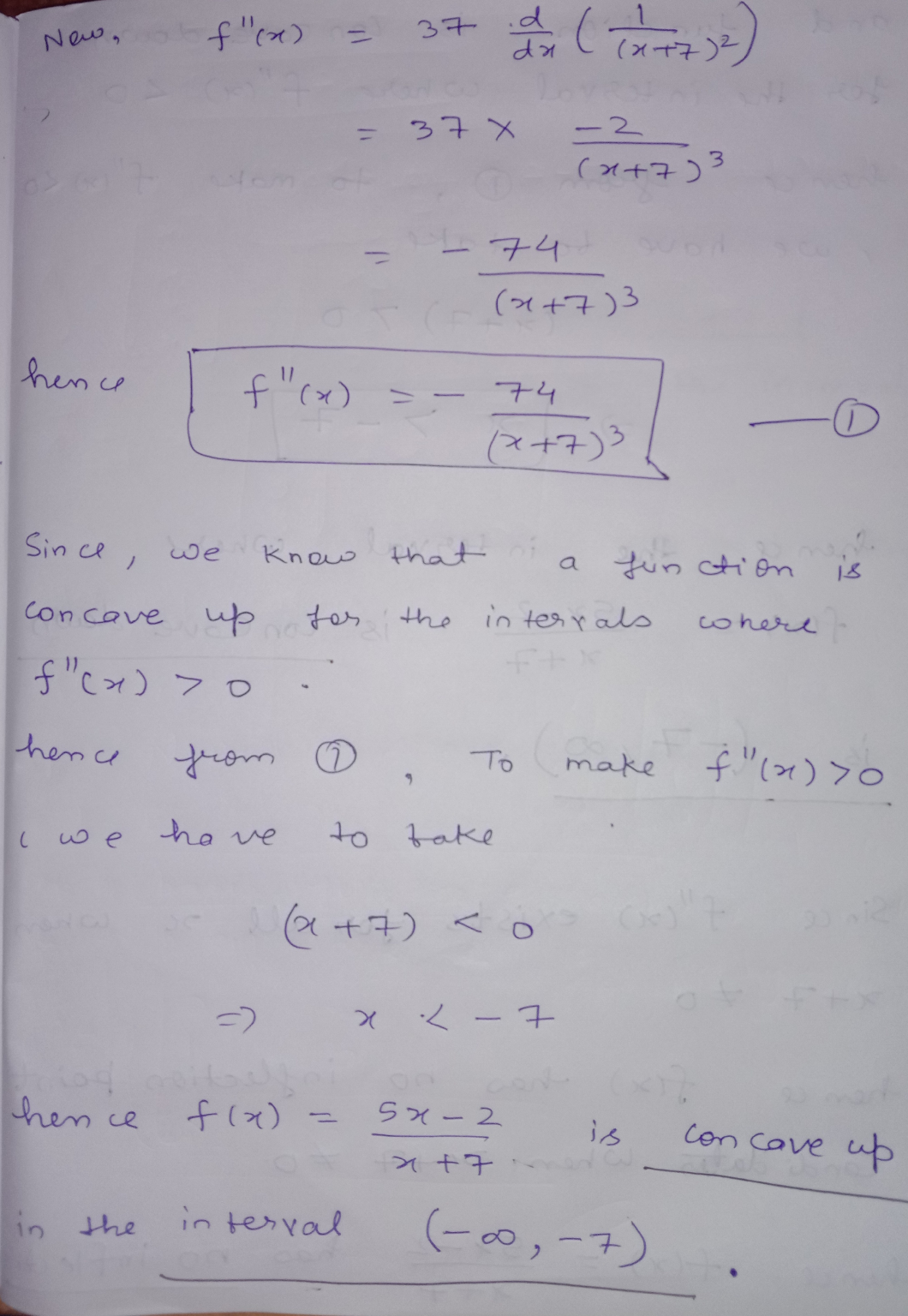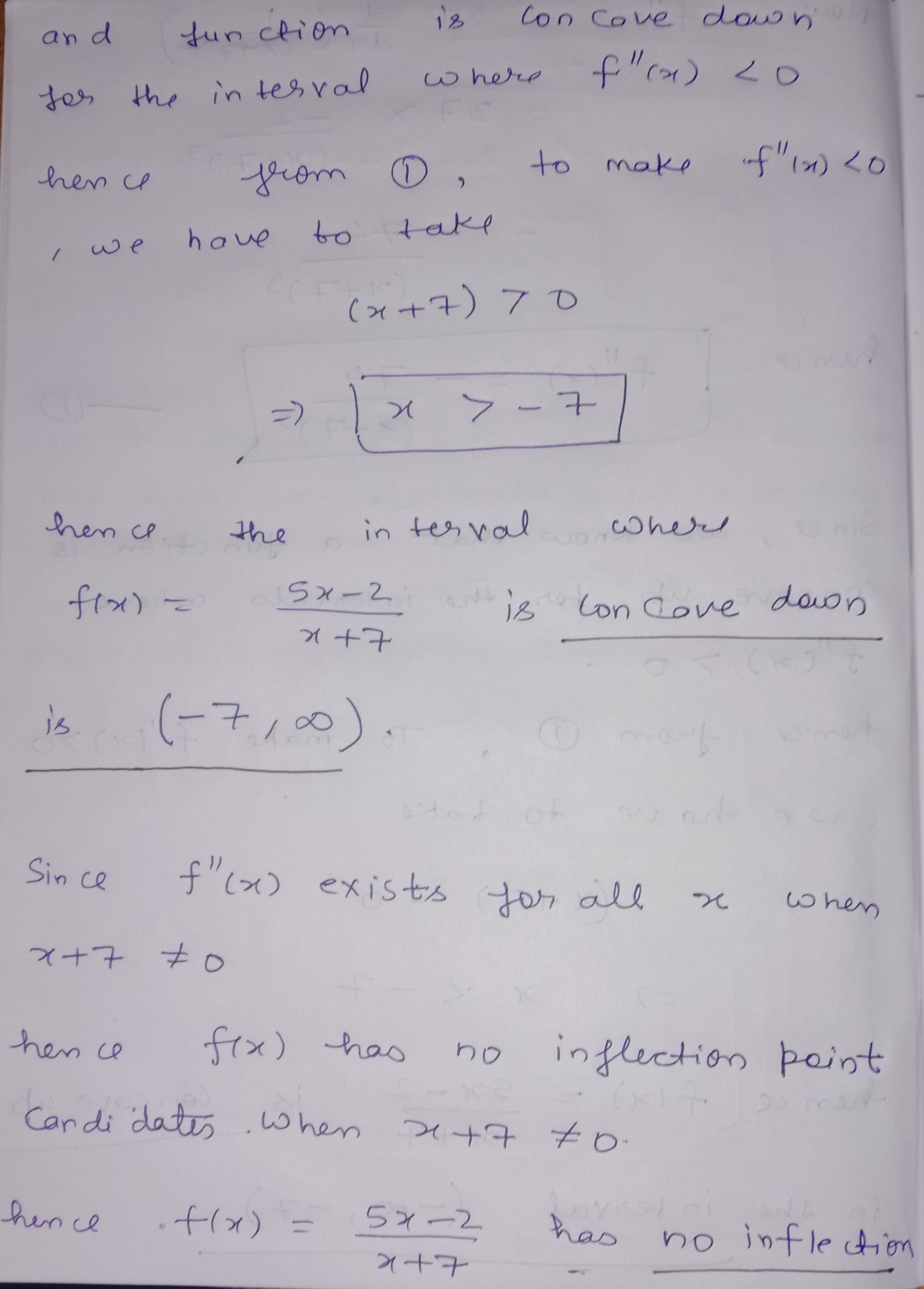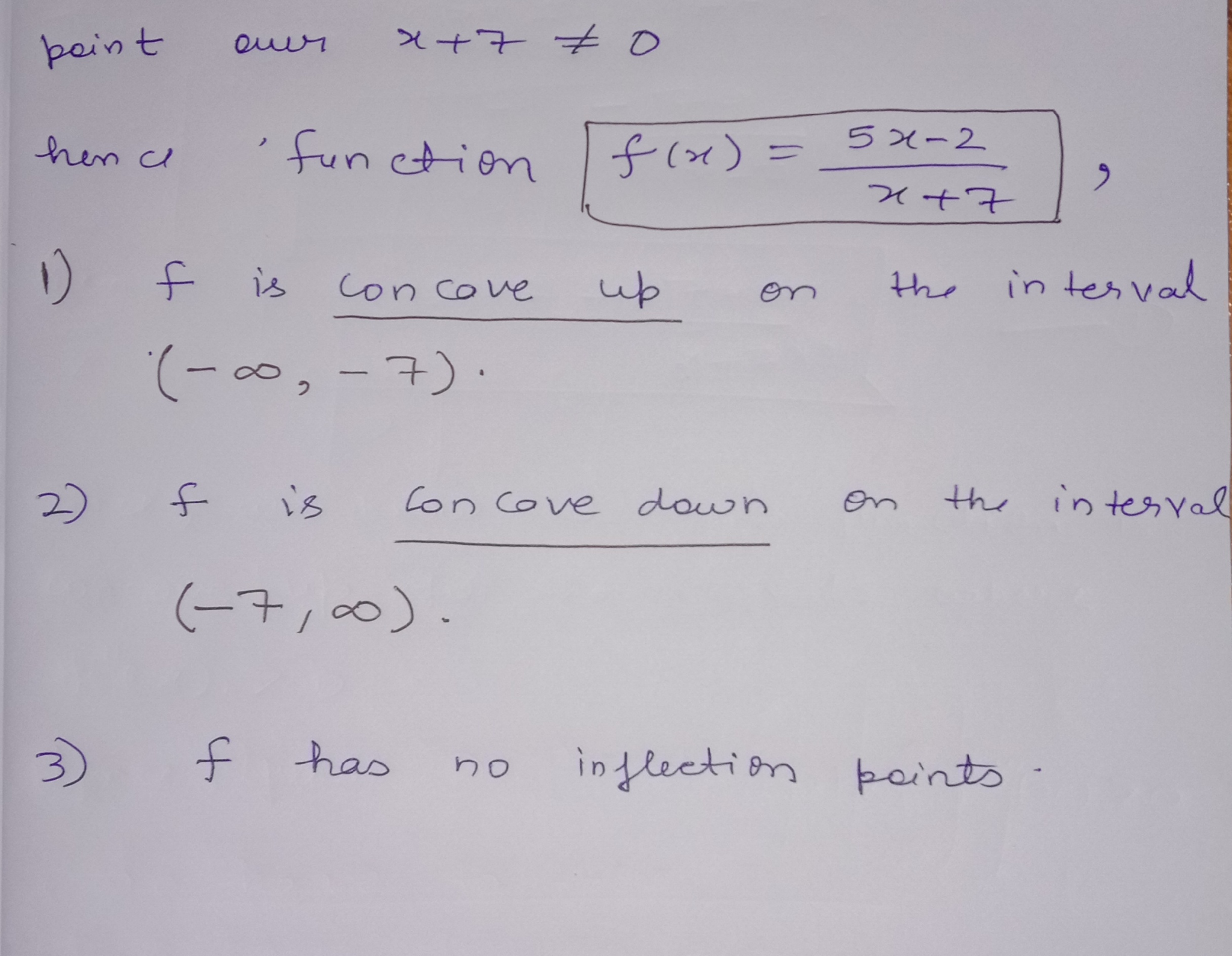#### Earn Coin

Coins can be redeemed for fabulous gifts.

Similar Homework Help Questions
• ### Let f(x)=3x-7/x+2. Find the open intervals on which f is concave up (down). Then determine the x-coordinates of all infl...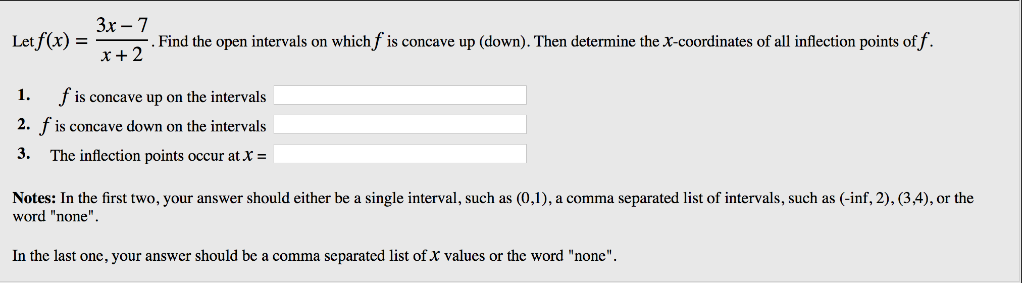Let f(x)=3x-7/x+2. Find the open intervals on which f is concave up (down). Then determine the x-coordinates of all inflection points of f. Let Find the open intervals on which is concave up down Then determine the X-coordinates of all inflection points of x = f is concave up on the intervals 1. 2. f is concave down on the intervals 3· The inflection points occur at Notes: In the first two, your answer should either be a single interval,...

• ### Homework14: Problem 13 Previous Problem Problem List Next Poblem (1 point) Let f(x) = – 4...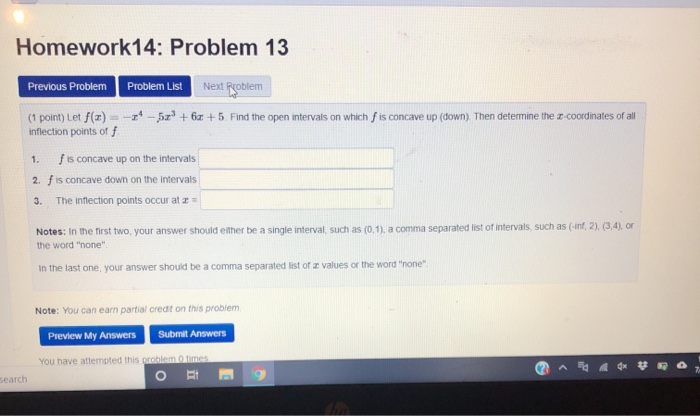Homework14: Problem 13 Previous Problem Problem List Next Poblem (1 point) Let f(x) = – 4 – 579 +6 +5. Find the open intervals on which fis concave up (down) Then determine the so-coordinates of all inflection points off f is concave up on the intervals 2. f is concave down on the intervals The inflection points occur at 1. 3. Notes: In the first two your answer should either be a single interval, such as (0,1), a comma separated...

• ### 1. (1 point) Let f(x) = -3 - 9x? +152 +5. Find the open intervals on...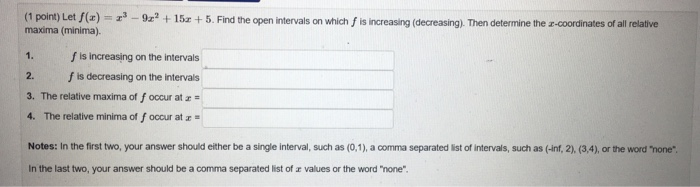1. (1 point) Let f(x) = -3 - 9x? +152 +5. Find the open intervals on which is increasing (decreasing). Then determine the 2-coordinates of all relative maxima (minima). f is increasing on the intervals f is decreasing on the intervals 3. The relative maxima of foccur at 2 = 4. The relative minima off occur at - 2. Notes: In the first two your answer should either be a single interval, such as (0,1), a comma separated list of...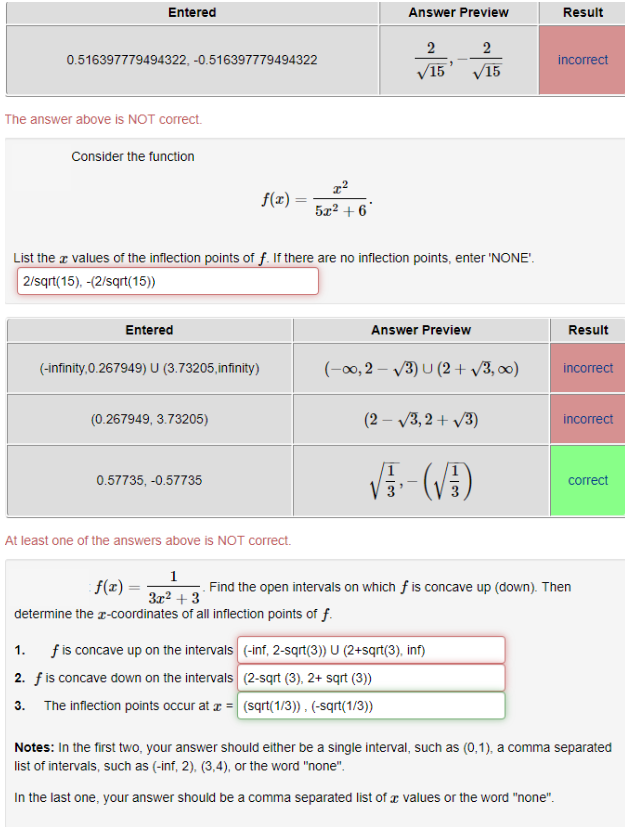Consider the function...... Please help for all incorrect answers Result Entered Answer Preview 0.516397779494322, -0.516397779494322 incorrect 15' 15 The answer above is NOT correct Consider the function 5r2 + List the r values of the inflection points of f. If there are no inflection points, enter 'NONE 2/sqrt(15), -(2/sqrt(15)) Entered Answer Preview Result (-0o, 2- 3) U (2 3,oo) incorrect (-infinity,0.267949) U (3.73205,infinity) (2- V3,2+ v3) (0.267949, 3.73205) incorrect 0.57735,-0.57735 correct At least one of the answers above is NOT...

• ### need help with these.please. I-8 Let f(2) Find the open intervals on which fis increasing (decreasing)....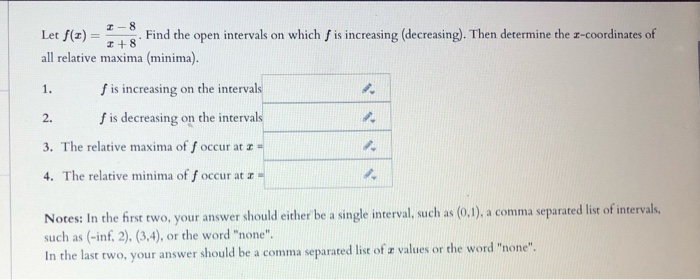need help with these.please. I-8 Let f(2) Find the open intervals on which fis increasing (decreasing). Then determine the x-coordinates of +8 all relative maxima (minima). 1. f is increasing on the intervals 2. fis decreasing on the intervals 3. The relative maxima of f occur at - 4. The relative minima off occur at 2 - Notes: In the first two your answer should either be a single interval, such as (0,1), a comma separated list of intervals, such...

• ### Determine the intervals on which the given function is concave up or down and find the...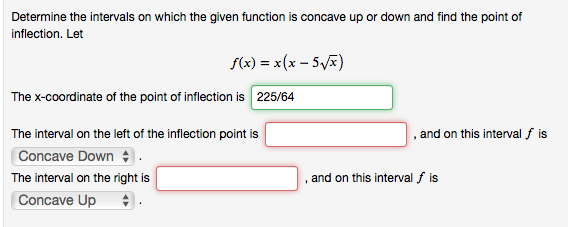Determine the intervals on which the given function is concave up or down and find the point of inflection. Let f(x) = x(x - 5) The x-coordinate of the point of inflection is 225/64 , and on this interval f is The interval on the left of the inflection point is Concave Down The interval on the right is Concave Up and on this interval f is Determine the intervals on which the given function is concave up or down...

• ### Find the largest open intervals on which the function is concave upward or concave downward, and...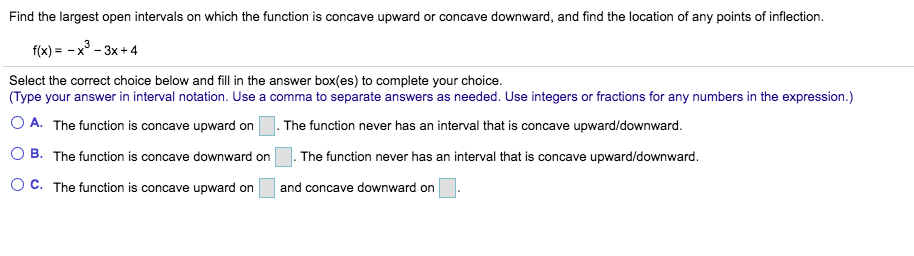Find the largest open intervals on which the function is concave upward or concave downward, and find the location of any points of inflection. f(x) = -x - = -x - 3x +4 Select the correct choice below and fill in the answer box(es) to complete your choice. (Type your answer in interval notation. Use a comma to separate answers as needed. Use integers or fractions for any numbers in the expression.) O A. The function is concave upward on...

• ### Determine the intervals on which the following function is concave up or concave down. Identify any...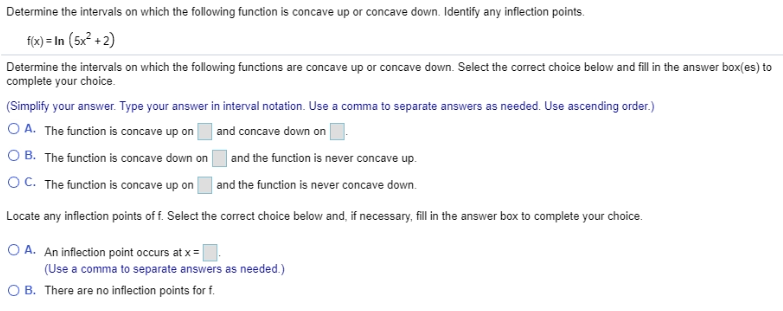Determine the intervals on which the following function is concave up or concave down. Identify any inflection points. f(x) = In (5x2 + 2) Determine the intervals on which the following functions are concave up or concave down. Select the correct choice below and fill in the answer box(es) to complete your choice (Simplify your answer. Type your answer in interval notation. Use a comma to separate answers as needed. Use ascending order.) O A. The function is concave up...

• ### D the open intervals where the function is concave Select the correct choice ward or concave down...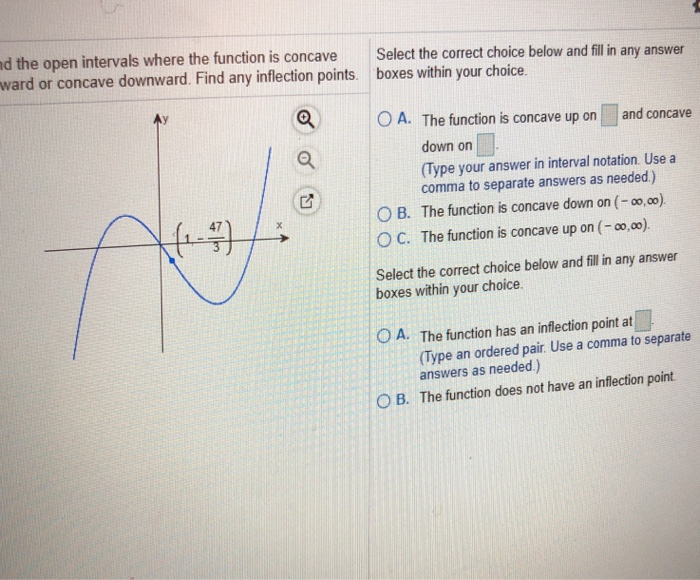d the open intervals where the function is concave Select the correct choice ward or concave downward. Find any inflection points boxes within your choice below and fill in any answer O A. The function is concave up on and concave down on (Type your answer in interval notation. Use a comma to separate answers as needed) O B. The function is concave down on (-o,00) ° C. The function is concave up on (-00,00) 47 Select the correct choice...

• ### Find the maximum and minimum values of the function g(0) interval [o. 7 2θ-4 sin(θ) on the Preview Minimum value-pi/3+2pi Maximum value O Preview Given the function f(z) = 2e - List the x-coordinates...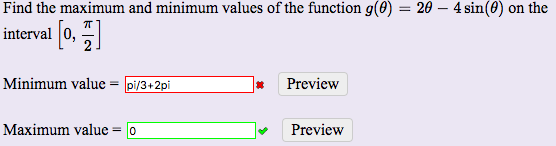Find the maximum and minimum values of the function g(0) interval [o. 7 2θ-4 sin(θ) on the Preview Minimum value-pi/3+2pi Maximum value O Preview Given the function f(z) = 2e - List the x-coordinates of the critical values (enter DNE if none) DNE List the x-coordinates of the inflection points (enter DNE if none) DNE List the intervals over which the function is increasing or decreasing (use DNE for any empty intervals) Increasing on DNE Preview Decreasing on -1/5 *Preview...

Free Homework App